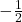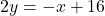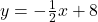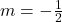## write the slope of x+2y=16 pls help fast

Question

write the slope of x+2y=16 pls help fast

in progress 0
3 weeks 2021-08-26T05:03:46+00:00 2 Answers 0 views 0

1. Step-by-step explanation:

x+2y=16

-× -×

2y=-x+16

÷2. ÷2

y=-1/2x+8

slope is -1/2

2. Answer:Step-by-step explanation:

To find the slope, let’s turn the equation into slope-interept form. In slope intercept form, y=mx+b, we know that m=slope.[subtract both sides by x][divide both sides by 2]Now, we know that. That tells usis the slope.